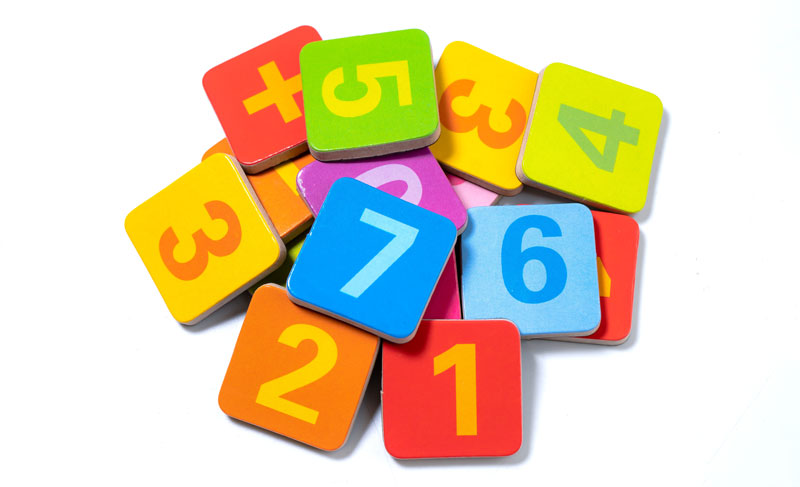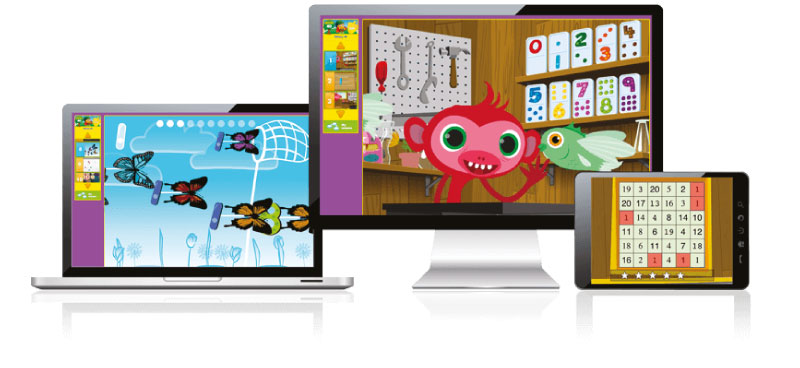# 15 Interesting Math Trivia – Questions and Answers

Looking to have some fun with numbers? Math doesn’t always have to be boring. The next time your mate at school tricks you into a Math trivia question, be prepared to answer it with confidence. We have compiled a list of interesting brain-wrecking Math Quiz questions & answers for you to memorize.#1 Is Zillion a real number?

Many get confused about whether zillion is an actual number or just a made-up figure. It rhymes just like million, trillion, and billion, and hence zillion sounds like an actual number. However, zillion is just an informal term to address an enormous or indefinite number. Zillion isn’t actually a number.

#2 How many configurations does a Rubik’s Cube have?

The famous Rubik’s cube is an iconic puzzle toy, and many children struggle to find a correct solution, often feeling frustrated. It’s pretty enthralling to solve this messy-looking colorful cuboid. After a few years of trial & error, it was discovered that the cube can be solved in as many as 20 moves and there are about 43 quintillion possible configurations of the Cube. Wow, that gives us enough motivation to pick up one and get cracking!

#3 What is the longest side of the right-angle triangle called?

The hypotenuse is the longest side of a right-angle triangle and it is always the side opposite to the right angle itself.

#4 Who was considered the fastest human calculator?

Scott Flansburg is a Guinness World Record Holder (2001) for the fastest mental calculation after adding the same number to itself 36 times in 15 seconds.

#5 Which number is spelt with letters in descending order?

‘One’ is the only number that is spelt with the letters arranged in descending order.

#6 What is the smallest perfect number?

A perfect number is a positive integer that is equal to the sum of its proper divisors. Number ‘6’ is the smallest perfect number, as the sum of 1, 2, and 3, together make 6.

#7 Which number is a ‘magic number’?

Number ‘9’ is considered as a magic number, as the sum of the digits of the multiples of 9, is always 9. For example; (18) 8 + 1 = 9, (54) 5 + 4 = 9, (81) 8 + 1 = 9.

#8 How many colors are needed on a map to ensure that no 2 colors share the same border?

In mathematics, the four-color theorem states that you only require four colors to depict the regions on a map, such that no two adjacent areas share the same color.

#9 Is -40 degrees Celsius equivalent to -40 degrees Fahrenheit?

True. Though Celsius and Fahrenheit are two different temperature scales, -40 always shows the same measurement in °C and °F.

#10 Is Pi a rational or irrational number?

Pi is called an infinite decimal and it’s a real number that cannot be expressed in a simple fraction.

#11 What is zero to the power of zero?

Any number to the power of zero is 1, so (30 = 1) and zero to the power of any number is always zero (02 =0). But when it comes to zero to the power of zero, the answer is undefined (00 =??).

#12 When two circles have the same centre, what is it called?

Concentric circles are circles with a common center.

#13 When something is going to occur, which mathematical term is used to express the likelihood of the event?

‘Probability’ is the extent to which something is likely to happen or be the case.

#14 The number hundred is derived from the ancient word, “hundrath,” which actually means what?

The word “hundred” comes from the old Norse term, “hundrath”, which actually means 120.

#15 Which number has the most synonyms?

‘Zero’, which can also be addressed as; nil, nada, zilch, zip, nought, and many more.Mathseeds is an euphoric online Math program that caters to children between ages 3 to 9 years. Schools in Dubai and Middle East Regions, promote Mathseeds and even include activities from this platform into their lesson-plan, to make the session more interesting for kids. The activities are carefully created by educational experts and hence ensures age-appropriateness. It even showcases the Mathematical progress of children and they are encouraged to create a custom Avatar – to keep themselves motivated. Mathseeds availability in the UAE and other GCC regions.